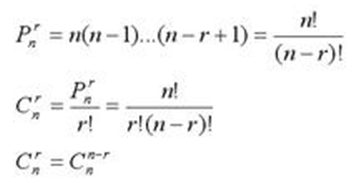排列 P------和顺序有关

组合 C -------不牵涉到顺序的问题

排列分顺序，组合不分

例如

把5本不同的书分给3个人，有几种分法. “排列”

把5本书分给3个人，有几种分法“组合”

1．排列及计算公式

从 n 个不同元素中，任取 m(m≤n)个元素按照一定的顺序排成一列，叫做从 n 个不同元素中取出 m 个元素的一个排列；从 n 个不同元素中取出 m(m≤n)个元素的所有排列的个数，叫做从 n 个不同元素中取出 m 个元素的排列数，用符号 p(n，m)表示.

p(n，m)=n(n-1)(n-2)……(n-m+1)= n！/(n-m)！(规定 0！=1).

2．组合及计算公式

从 n 个不同元素中，任取 m(m≤n)个元素并成一组，叫做从 n 个不同元素中取出 m 个元素的一个组合；从 n 个不同元素中取出 m(m≤n)个元素的所有组合的个数，叫做从 n 个不同元素中取出 m 个元素的组合数.用符号

c(n，m) 表示.

c(n，m)=p(n，m)/m！=n！/((n-m)！*m！)；c(n，m)=c(n，n-m)；

3．其他排列与组合公式

从 n 个元素中取出 r 个元素的循环排列数＝p(n，r)/r=n！/r(n-r)！.

n 个元素被分成 k 类，每类的个数分别是 n1，n2，...nk 这 n 个元素的全排列数为n！/(n1！*n2！*...*nk！).

k 类元素，每类的个数无限，从中取出 m 个元素的组合数为 c(m+k-1，m).

排列（Pnm(n 为下标，m 为上标)）

Pnm=n×（n-1）....（n-m+1）；Pnm=n！/（n-m）！（注：！是阶乘符号）；Pnn（两个 n分别为上标和下标） =n！；0！=1；Pn1（n 为下标 1 为上标）=n

组合（Cnm(n 为下标，m 为上标)）

Cnm=Pnm/Pmm ；Cnm=n！/m！（n-m）！；Cnn（两个 n 分别为上标和下标） =1 ；

Cn1（n 为下标 1 为上标）=n；Cnm=Cnn-m公式P是指排列，从N个元素取R个进行排列。

公式C是指组合，从N个元素取R个，不进行排列。

N-元素的总个数

R参与选择的元素个数

！-阶乘 ，如    9！＝9*8*7*6*5*4*3*2*1

从N倒数r个，表达式应该为n*（n-1)*(n-2)..(n-r+1)；

因为从n到（n-r+1)个数为n－（n-r+1)＝r